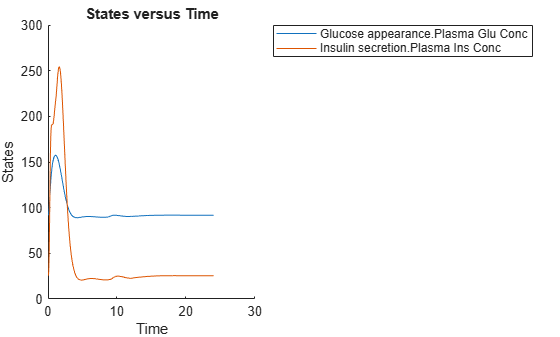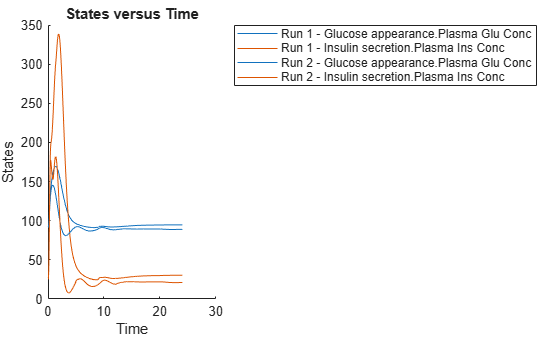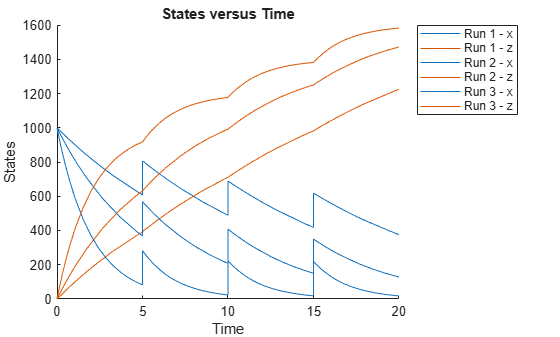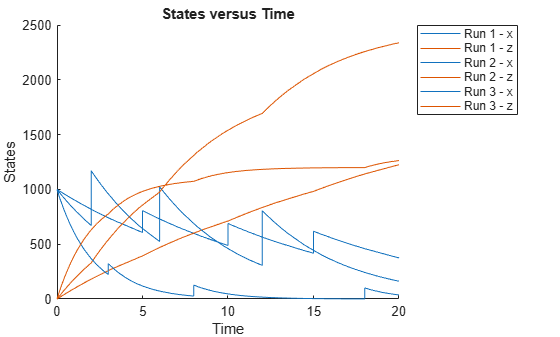Main Content

# SimFunction object

Function-like interface to execute SimBiology models

## Description

The `SimFunction` object provides an interface that allows you to execute a SimBiology® model like a function and a workflow to perform parameter scans (in parallel if Parallel Computing Toolbox™ is available), Monte Carlo simulations, and scans with multiple or vectorized doses. Since a `SimFunction` object can be executed like a function handle, you can customize it to integrate SimBiology models with other MATLAB® products and other custom analyses (such as visual predictive checks).

Use the `createSimFunction` method to construct the SimFunction object. SimFunction objects are immutable once created and automatically accelerated at the first function execution.

## Syntax

If you specified any dosing information when you called `createSimFunction` to construct the `SimFunction` object `F`, then `F` has the following syntaxes.

`simdata = F(phi,t_stop,u,t_output)` returns a `SimData object` `simdata` after simulating a SimBiology model using the initial conditions or simulation scenarios specified in `phi`, simulation stop time, `t_stop`, dosing information, `u`, and output time, `t_output`.

`simdata = F(phi,t_stop,u)` runs simulations using the input arguments `phi`, `t_stop`, and `u`.

If you did not specify any dosing information when you called `createSimFunction`, then `F` has the following syntaxes:

`simdata = F(phi,t_stop)` returns a `SimData object` `simdata` after simulating the model using initial conditions or simulation scenarios specified in `phi`, and simulation stop time, `t_stop`.

`simdata = F(phi,t_stop,[],t_output)` uses the input arguments `phi`, `t_stop`, empty dosed argument `[]`, and `t_output`. You must specify `u`, the dosing information, as an empty array`[]` for this signature. When `t_output` is empty and `t_stop` is specified, the simulations report the solver time points until `t_stop`. When `t_output` is specified and `t_stop` is empty, only the time points in `t_output` are reported. When both are specified, the reported time points are the union of solver time points and the time points in `t_output`. If the last `t_output` is greater than the corresponding `t_stop`, then simulation proceeds until the last time point in `t_output`.

````simdata = F(phi,tbl)` uses the input arguments `phi` and `tbl`. Using this signature only lets you specify output times as one of the variables of `tbl`. Any data row in `tbl` where all dependent variable columns having `NaN` values is ignored.```[T,Y] = F(_)``` returns `T`, a cell array of numeric vector, and `Y`, a cell array of 2-D numeric matrices, using any of the input arguments in the preceding syntaxes.```

## Input Arguments

 `phi` One of the following: Empty array `[]` or empty cell array `{}`, meaning to perform simulations using the baseline initial values, that is, the values listed in the `Parameter` property of the `SimFunction` object, without altering them.Matrix of size S-by-P, where S is the number of simulations to perform and P is the number of parameters specified in the `params` argument when you called `createSimFunction` to construct `F`. Each simulation is performed with the parameters specified in the corresponding row of `phi`.S-by-V matrix of variant objects or a cell column vector of length S, where each element consists of a row vector of variant objects. S is the number of simulations to perform, and V is the number of variant objects. These variants are only allowed to modify the `SimFunction` input parameters, that is, model elements that were specified as the `params` input argument when you called `createSimFunction`. In other words, you must specify the variant parameters as the input parameters when you create the `SimFunction` object. Any `SimFunction` input parameters that are not specified in the variants use their baseline initial values.If, within a row of variants, multiple entries refer to the same model element, the last occurrence is used for simulation.Scalar `SimBiology.Scenarios` object containing S number of scenarios. When `phi` is specified as a `1`-by-P or `1`-by-V matrix (or a `Scenarios` object with only one scenario), then all simulations use the same parameters, and the number of simulations is determined from the `t_stop`, `u`, or `t_output` argument in that order. For example, if `phi` and `t_stop` have a single row and `u` is a matrix of size N-by-DoseTargets, the number of simulations is determined as N. When `phi` is specified as a `SimBiology.Scenarios` object, all scenarios are simulated. Variants are applied before values from the scenarios are set. `t_stop` Scalar specifying the same stop time for all simulationsVector of size N specifying a stop time for each simulation for all N simulations `u` Empty array `[]` to apply no doses during simulation unless you specify `phi` as a `Scenarios` object that has doses defined in its entries. `table` of dosing information with two or three variables containing `ScheduleDose` data (`ScheduleDose` table), namely, dose time, dose amount, and dose rate (optional). Name the table variables as follows.`u.Properties.VariableNames = {'Time','Amount','Rate'};` If `UnitConversion` is on, specify units for each variable. For instance, you can specify units as follows.`u.Properties.VariableUnits = {'second','molecule','molecule/second'};`This table can have multiple rows, where each row represents a dose applied to the dose target at a specified dose time with a specified amount and rate if available.`table` with one row and five variables containing `RepeatDose` data (`RepeatDose` table). Dose rate variable is optional. Name the variables as follows.`u.Properties.VariableNames = {'StartTime','Amount','Rate','Interval','RepeatCount'};` If `UnitConversion` is on, specify units for each variable. Units for `'RepeatCount'` variable can be empty `''` or `'dimensionless'`. The unit of the `'Amount'` variable must be dimensionally consistent with that of the target species. For example, if the unit of target species is in an amount unit (such as mole or molecule), then the `'Amount'` variable unit must have the same dimension, i.e., its unit must be an amount unit and cannot be a mass unit (such as gram or kilogram). The unit for the `'Rate'` variable must be dimensionally consistent as well.`u.Properties.VariableUnits = {'second','molecule','molecule/second','second','dimensionless'};`TipIf you already have a dose object (`ScheduleDose` or `RepeatDose`), you can get this dose table by using the `getTable` method of the object.Cell array of tables of size 1-by-N, where N is the number of dose targets. Each cell can represent either table as described previously.Cell array of tables of size S-by-N, where S is the number of simulations and N is the number of dose targets. Each cell represents a table. S is equal to the number of rows in `phi`. If `u` is a cell array of tables, then: If `phi` is also a `Scenarios` object, the combined number of doses in the `Scenarios` object and the number of columns in `u` must equal to the number of elements in the `Dosed` property of the `SimFunction` object. In other words, the dosing information that you specified during the creation of the `SimFunction` object must be consistent with the dosing information you specify in the execution of the object. The total number of elements for the `Dosed` property is equal to the combination of any doses from the input `Scenarios` object and doses in the dosed input argument of `createSimFunction`.If `phi` is not a `Scenarios` object, the number of columns (N) in the cell array `u` must be equal to the number of elements in the `Dosed` property of the `SimFunction` object. The order of dose tables must also match the order of dosed species in `createSimFunction`. That is, SimBiology assumes one-to-one correspondence between the columns of `u` and dose targets specified in the `Dosed` property of the `SimFunction` object, meaning the doses (dose tables) in the first column of `u` are applied to the first dose target in the `Dosed` property and so on. The ith dose for the jth dose target is ignored if `u{i,j} = []`.If the ith dose is not parameterized, `u{i,j}` can be `[]` or either type of table (the `ScheduleDose` or `RepeatDose` table).If the ith dose is parameterized, `u{i,j}` must be `[]` or a `RepeatDose` table with one row and a column for each property (`StartTime`, `Amount`, `Rate`, `Interval`, `RepeatCount`) that is not parameterized. It is not required to create a column for a dose property that is parameterized. If all of the properties are parameterized, you can pass in a table with one row and no columns to specify the parameterized dose is applied during simulations. To create such table, use `table.empty(1,0)`. `t_output` Vector of monotonically increasing output times that is applied to all simulationsCell array containing a single time vector that is applied to all simulationsCell array of vectors representing output times. The ith cell element provides the output times for the ith simulation. The number of elements in the cell array must match the number of rows (simulations) in `phi`. `tbl` `table` or `dataset` (Statistics and Machine Learning Toolbox) that has time and dosing information such as group labels, independent variable, dependent variable(s), amount(s), and rate(s). You must name the variables of the table or data set as `'GROUP','TIME','DEPENDENTVAR1','DEPENDENTVAR2',...,'AMOUNT1','RATE1','AMOUNT2','RATE2',...`. The rate variable is optional for each dose. If the `Dosed` property of the SimFunction object `F` is empty, then amount- and rate-related variables are not required. The number of groups in `tbl` must be equal to the number of rows, or the number of scenarios, in `phi`. The combined dosing information in `phi`, if `phi` is a `SimBiology.Scenarios` object, and the number of amount and rate columns in `tbl` must be equal to the number of doses in the `Dosed` property of the object `F`. If `tbl` has additional columns, they are ignored. If `UnitConversion` is on, specify a unit for each variable. The unit of `'Amount'` variable must be dimensionally consistent with that of the target species. See the description of the input argument `u` for details.

## Output Arguments

 `simdata` Array of SimData objects that contains results from executing the SimFunction `F`. The number of elements in the `simdata` array is the same as the number of rows in `phi`. The number of columns in each element of the `simdata` array, that is, `simdata(i).Data`, is equal to the number of elements in the `observed` cell array which was specified when creating `F`. `T` Cell array containing a numeric vector of size ```S x 1```. `S` is the number of simulations. The ith element of T contains the time point from the ith simulation. `Y` Cell array of 2-D numeric matrices. The ith element of `Y` contains data from the ith simulation. The number of rows in `T{i}` is equal to the number of rows in `Y{i}`.

## Constructor Summary

 createSimFunction (model) Create SimFunction object

## Method Summary

 accelerate(SimFunction) Prepare SimFunction object for accelerated simulations isAccelerated(SimFunction) Determine if SimFunction object is accelerated

## Property Summary

`Parameters`

`table` with variables named:

• `'Name'`

• `'Value'`

• `'Type'`

• `'Units'` (only if `UnitConversion` is turned on)

The table contains information about model quantities (species, compartments, or parameters) that define the inputs of a ```SimFunction object```. For instance, this table can contain parameters or species whose values are being scanned by the ```SimFunction object```. This property is read only.

`Observables`

`table` with variables named:

• `'Name'`

• `'Type'`

• `'Units'` (only if `UnitConversion` is turned on)

This table contains information about model quantities (species, compartments, or parameters) that define the output of a ```SimFunction object```. This property is read only.

`Dosed`

`table` containing dosing information with variables named:

• `'TargetName'`

• `'TargetDimension'` (only if `UnitConversion` is turned on)

In addition, the table also contains variables for each property that is parameterized. For each parameterized property, two variables are added to this table. The first variable has the same name as the property name and the value is the name of the specified parameter. The second variable has the property name suffixed by Value (PropertyNameValue), and the value is the default value of the parameter. If the `UnitConversion` is on, the unit column is also added with the name PropertyNameUnits.

Suppose the `Amount` property of a repeat dose targeting the Drug species is parameterized by setting it to a model parameter called `AmountParam` with the value of `10` milligram, and `UnitConversion` is on. The `Dosed` table contains the following variables:

TargetNameTargetDimensionAmountAmountValueAmountUnits
`'Drug'``'Mass (e.g., gram)' ``'AmountParam'`10`'milligram'`
`UseParallel`

Logical. If `true` and Parallel Computing Toolbox is available, SimFunction is executed in parallel. This property is read-only.

`UnitConversion`

Logical. If true:

• During the execution of the `SimFunction` object, `phi` is assumed to be in the same units as units for corresponding model quantities specified in the `params` argument when the object was created using the `createSimFunction` method.

• Time (`t_output` or `t_stop`) is assumed to be in the same unit as the `TimeUnits` property of the active `configset object` of the SimBiology model from which `F` was created.

• Variables of dose tables (`u`) must have units specified by setting `u.Properties.VariableUnits` to a cell array of appropriate units. The dimension of the dose target such as an amount (molecule, mole, etc.) or mass (gram, kilogram, etc.), is stored on the `Dosed` property of `F`.

• The simulation result is in the same units as those specified on the corresponding quantities in the SimBiology model from which `F` was created.

This property is read only.

`AutoAccelerate`

Logical. When true, the model is accelerated on the first evaluation of the `SimFunction` object.

This property is read only.

`DependentFiles`

Cell array of character vectors containing the names of files that the model depends on. This property is used for deployment. This property is read only.

## Examples

collapse all

This example shows how to simulate the glucose-insulin responses for the normal and diabetic subjects.

Load the model of glucose-insulin response. For details about the model, see the Background section in Simulating the Glucose-Insulin Response.

`sbioloadproject('insulindemo', 'm1')`

The model contains different initial conditions stored in various variants.

`variants = getvariant(m1);`

Get the initial conditions for the type 2 diabetic patient.

`type2 = variants(1)`
```type2 = SimBiology Variant - Type 2 diabetic (inactive) ContentIndex: Type: Name: Property: Value: 1 parameter Plasma Volume ... Value 1.49 2 parameter k1 Value 0.042 3 parameter k2 Value 0.071 4 parameter Plasma Volume ... Value 0.04 5 parameter m1 Value 0.379 6 parameter m2 Value 0.673 7 parameter m4 Value 0.269 8 parameter m5 Value 0.0526 9 parameter m6 Value 0.8118 10 parameter Hepatic Extrac... Value 0.6 11 parameter kmax Value 0.0465 12 parameter kmin Value 0.0076 13 parameter kabs Value 0.023 14 parameter kgri Value 0.0465 15 parameter f Value 0.9 16 parameter a Value 6e-05 17 parameter b Value 0.68 18 parameter c Value 0.00023 19 parameter d Value 0.09 20 parameter Stomach Glu Af... Value 125 21 parameter kp1 Value 3.09 22 parameter kp2 Value 0.0007 23 parameter kp3 Value 0.005 24 parameter kp4 Value 0.0786 25 parameter ki Value 0.0066 26 parameter [Ins Ind Glu U... Value 1 27 parameter Vm0 Value 4.65 28 parameter Vmx Value 0.034 29 parameter Km Value 466.21 30 parameter p2U Value 0.084 31 parameter K Value 0.99 32 parameter alpha Value 0.013 33 parameter beta Value 0.05 34 parameter gamma Value 0.5 35 parameter ke1 Value 0.0007 36 parameter ke2 Value 269 37 parameter Basal Plasma G... Value 164.18 38 parameter Basal Plasma I... Value 54.81 ```

Suppress an informational warning that is issued during simulations.

`warnSettings = warning('off','SimBiology:DimAnalysisNotDone_MatlabFcn_Dimensionless');`

Create SimFunction objects to simulate the glucose-insulin response for the normal and diabetic subjects.

• Specify an empty array `{}` for the second input argument to denote that the model will be simulated using the base parameter values (that is, no parameter scanning will be performed).

• Specify the plasma glucose and insulin concentrations as responses (outputs of the function to be plotted).

• Specify the species `Dose` as the dosed species. This species represents the initial concentration of glucose at the start of the simulation.

```normSim = createSimFunction(m1,{},... {'[Plasma Glu Conc]','[Plasma Ins Conc]'},'Dose')```
```normSim = SimFunction Parameters: Observables: Name Type Units _____________________ ___________ _______________________ {'[Plasma Glu Conc]'} {'species'} {'milligram/deciliter'} {'[Plasma Ins Conc]'} {'species'} {'picomole/liter' } Dosed: TargetName TargetDimension __________ _____________________ {'Dose'} {'Mass (e.g., gram)'} ```

For the diabetic patient, specify the initial conditions using the variant `type2`.

```diabSim = createSimFunction(m1,{},... {'[Plasma Glu Conc]','[Plasma Ins Conc]'},'Dose',type2)```
```diabSim = SimFunction Parameters: Observables: Name Type Units _____________________ ___________ _______________________ {'[Plasma Glu Conc]'} {'species'} {'milligram/deciliter'} {'[Plasma Ins Conc]'} {'species'} {'picomole/liter' } Dosed: TargetName TargetDimension __________ _____________________ {'Dose'} {'Mass (e.g., gram)'} ```

Select a dose that represents a single meal of 78 grams of glucose at the start of the simulation.

`singleMeal = sbioselect(m1,'Name','Single Meal');`

Convert the dosing information to the table format.

`mealTable = getTable(singleMeal);`

Simulate the glucose-insulin response for a normal subject for 24 hours.

`sbioplot(normSim([],24,mealTable));`Simulate the glucose-insulin response for a diabetic subject for 24 hours.

`sbioplot(diabSim([],24,mealTable));`Perform a scan using variants

Suppose you want to perform a parameter scan using an array of variants that contain different initial conditions for different insulin impairments. For example, the model `m1` has variants that correspond to the low insulin sensitivity and high insulin sensitivity. You can simulate the model for both conditions via a single call to the SimFunction object.

Select the variants to scan.

```varToScan = sbioselect(m1,'Name',... {'Low insulin sensitivity','High insulin sensitivity'});```

Check which model parameters are being stored in each variant.

`varToScan(1)`
```ans = SimBiology Variant - Low insulin sensitivity (inactive) ContentIndex: Type: Name: Property: Value: 1 parameter Vmx Value 0.0235 2 parameter kp3 Value 0.0045 ```
`varToScan(2)`
```ans = SimBiology Variant - High insulin sensitivity (inactive) ContentIndex: Type: Name: Property: Value: 1 parameter Vmx Value 0.094 2 parameter kp3 Value 0.018 ```

Both variants store alternate values for `Vmx` and `kp3` parameters. You need to specify them as input parameters when you create a SimFunction object.

Create a SimFunction object to scan the variants.

```variantScan = createSimFunction(m1,{'Vmx','kp3'},... {'[Plasma Glu Conc]','[Plasma Ins Conc]'},'Dose');```

Simulate the model and plot the results. `Run 1` include simulation results for the low insulin sensitivity and `Run 2` for the high insulin sensitivity.

`sbioplot(variantScan(varToScan,24,mealTable));`Low insulin sensitivity lead to increased and prolonged plasma glucose concentration.

Restore warning settings.

`warning(warnSettings);`

This example shows how to scan initial amounts of a species from a radioactive decay model with the first-order reaction $\frac{dz}{dt}=c\cdot x$, where `x` and `z` are species and `c` is the forward rate constant.

Load the sample project containing the radiodecay model `m1`.

`sbioloadproject radiodecay;`

Create a `SimFunction` object `f` to scan initial amounts of species `x`.

`f = createSimFunction(m1,{'x'},{'x','z'},[])`
```f = SimFunction Parameters: Name Value Type Units _____ _____ ___________ ____________ {'x'} 1000 {'species'} {'molecule'} Observables: Name Type Units _____ ___________ ____________ {'x'} {'species'} {'molecule'} {'z'} {'species'} {'molecule'} Dosed: None ```

Define four different initial amounts of species x for scanning. The number of rows indicates the total number of simulations, and each simulation uses the parameter value specified in each row of the vector.

`phi = [200; 400; 600; 800];`

Run simulations until the stop time is 20 and plot the simulation results.

`sbioplot(f(phi, 20));`This example shows how to simulate and scan a parameter of a radiodecay model while a species is being dosed.

Load the sample project containing the radiodecay model `m1`.

`sbioloadproject radiodecay;`

Create a `SimFunction` object `f` specifying parameter `Reaction1.c` to be scanned and species `x` as a dosed target.

`f = createSimFunction(m1,{'Reaction1.c'},{'x','z'},{'x'});`

Define a scalar dose of amount 200 molecules given at three time points (5, 10, and 15 seconds).

```dosetime = [5 10 15]; dose = [200 200 200]; u = table(dosetime', dose'); u.Properties.VariableNames = {'Time','Amount'}; u.Properties.VariableUnits = {'second','molecule'};```

Define the parameter values for `Reaction1.c` to scan.

`phi = [0.1 0.2 0.5]';`

Simulate the model for 20 seconds and plot the results.

`sbioplot(f(phi,20,u));`You can also specify different dose amounts at different times.

```d1 = table(5,100); d1.Properties.VariableNames = {'Time','Amount'}; d1.Properties.VariableUnits = {'second','molecule'}; d2 = table(10,300); d2.Properties.VariableNames = {'Time','Amount'}; d2.Properties.VariableUnits = {'second','molecule'}; d3 = table(15,600); d3.Properties.VariableNames = {'Time','Amount'}; d3.Properties.VariableUnits = {'second','molecule'};```

Simulate the model using these doses and plot the results.

`sbioplot(f(phi,20,{d1;d2;d3}));`You can also define a cell array of dose tables.

```u = cell(3,1); dosetime = [5 10 15]; dose = [200 200 200]; u{1} = table(dosetime',dose'); u{1}.Properties.VariableNames = {'Time','Amount'}; u{1}.Properties.VariableUnits = {'second','molecule'}; dosetime2 = [2 6 12]; dose2 = [500 500 500]; u{2} = table(dosetime2', dose2'); u{2}.Properties.VariableNames = {'Time','Amount'}; u{2}.Properties.VariableUnits = {'second','molecule'}; dosetime3 = [3 8 18]; dose3 = [100 100 100]; u{3} = table(dosetime3', dose3'); u{3}.Properties.VariableNames = {'Time','Amount'}; u{3}.Properties.VariableUnits = {'second','molecule'};```

Simulate the model using the dose tables and plot results.

`sbioplot(f(phi,20,u));`Gillespie, D.T. (1977). Exact Stochastic Simulation of Coupled Chemical Reactions. The Journal of Physical Chemistry. 81(25), 2340–2361.

## SupportGet trial now